Smartick is an online platform for children to master math in only 15 minutes a day

May29

# How to Evaluate Number Sequences

What are number sequences? How can we guess what number will come next? In this post, we’ll give you the guidelines that you need to solve number sequence problems.

Number sequences are numbers that are organized to follow a certain rule.

The hardest part is figuring out the rule, but once we have it, it’s only a matter of following it in order to determine the next numbers in the sequence.

We are going to go over the steps that can help us find out the rule of the number sequence.

The first step is to determine if the sequence is ascending, descending or a combination of both.

### Ascending Number Sequences:

Ascending number sequences are sequences where every number is greater than the one before it. These are the easiest to solve because ascending implies addition, multiplication or a combination of both methods.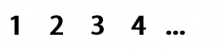This sequence is ascending and in order to move from one number to the next, we only need to add 1.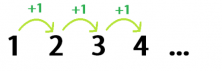This means that the next number of this sequence is 4 + 1 = 5

• Multiplication Number Sequences: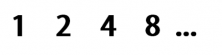This sequence also goes in ascending order but this time, in order to move from one number to the next, we have multiplied by 2.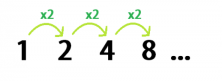This means that the next number of this sequence is 8 x 2 = 16

• Addition and Multiplication Number Sequences: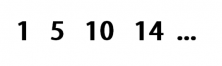This is another ascending order sequence, however, we aren’t adding one number nor multiplying by another. Instead, we are alternating between adding 4 and multiplying by 2.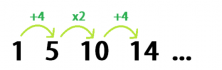After adding 4, multiplying by 2 and adding 4 again, the next step is multiplying by 2. This means that the result of this sequence is 14 x 2 = 28

### Descending Number Sequences:

Descending number sequences are sequences where every number is less than the one that comes before it. The mathematical operations that we need to use as rules are subtraction and division.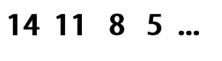This number sequence goes in descending order. To move from one number to the next, we subtract 3.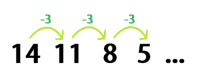This means that the next number in this sequence is 5 – 3 = 2.

To wrap up this week’s post, I’ll leave you with a sequence to see if you can figure out the rule.  Are you able to do it?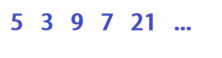If you think you’ve figured out the rule for this number sequence, leave a comment and share it with your friends and classmates. Also, if you want to practice these kinds of problems, and more, go to Smartick and try it for free!